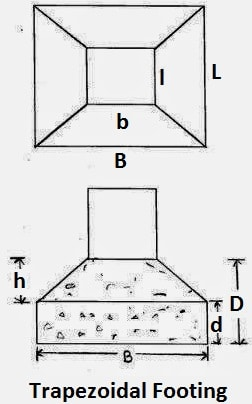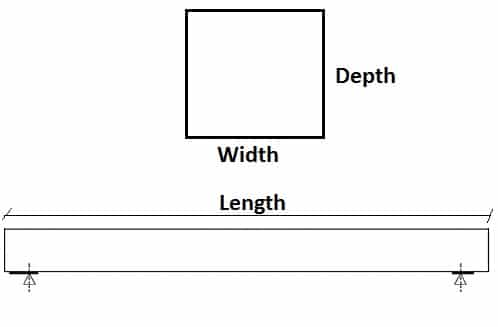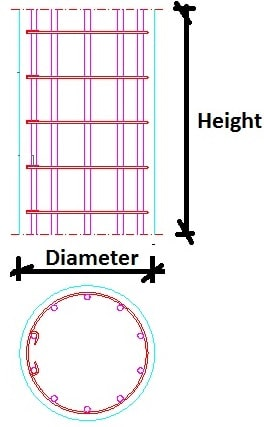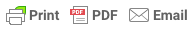Sorry, you do not have permission to ask a question, You must login to ask question. Become VIP Member

This Concrete Calculator calculates quantities of materials required for construction of concrete slabs, beams, columns, footings and trapezoidal footings in cubic meters and cubic feet.

The quantity of cement, sand and aggregates for various proportions of concrete such as M10 (1:3:6), M15 (1:2:4), M20 (1:1.5:3), M25(1:1:2) and other custom concrete mix can be calculated for shapes such as rectangular, square, circular, trapezoidal concrete members.

## Concrete Calculator

 Select Concrete Mix Proportion Cement : Sand : Aggregates : W/C Ratio :
 Select Concrete Member*:Select MemberSlabBeamRectangular FootingTrapezoidal FootingRectangular ColumnCircular ColumnSelect Unit System*:Select UnitsUnited States Standard FeetMetric

## Concrete Calculator – Results

Volume of Concrete = m3 = Cubic Yard = CFT = Pounds

Quantity of Cement = kg = Bags of 50 kg = Pounds& Ad Free!

GET VIP MEMBERSHIP NOW!

Quantity of Sand = kg = m3 = Cubic Yard = CFT = Pounds

Quantity of Coarse Aggregates = kg = m3 = Cubic Yard = CFT = Pounds

Quantity of Water = Liter = Gallons

Calculate Quantities of Materials for Concrete

Concrete – Definition, Grades, Components, Manufacture, Construction and Articles